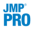Publication date: 07/30/2020

## Save Options

The Text Explorer red triangle menu contains the following options to save information to data tables, table columns, and column properties:

Save Document Term Matrix

Saves columns to the data table for each column of the document term matrix (up to a specified Maximum Number of Terms).Save Stacked DTM for Association

Saves a stacked version of the document-term matrix to a JMP data table. The stacked format is appropriate for analysis in the Association Analysis platform. See “Association Analysis” in Predictive and Specialized Modeling. If you specify an ID variable in the Text Explorer launch window, the ID variable is used to identify the rows that each term came from in the original text data table. The stacked table also contains a table script to launch Association Analysis.

Save DTM Formula

Saves a vector-valued formula column to the data table. The length of the vector depends on user-specified options for the maximum number of terms, the minimum term frequency, and the weighting. The resulting column uses the Text Score() JSL function. For more information about this function, see Help > Scripting Index.

Save Term Table

Creates a JMP data table that contains each term from the Term List, the number of occurrences, and the number of documents that contain each term. If you select the Score Terms by Column option after selecting Save Term Table, a column containing scores for each term is added to the data table created by the Save Term Table option.

Score Terms by Column

Saves scores based on values in a specified column to the JMP data table created by the Save Term Table option. The scores for each term are the mean value of the specified column weighted by the number of occurrences of the term in each row. If you have already selected the Save Term Table option, the Score Terms by Column option adds a column containing scores to the data table created by the Save Term Table option. Otherwise, the JMP data table for the term table is created. When the specified column is not Continuous, columns containing scores for each level in the specified column are created.

### Document Term Matrix Specifications Window

When you select the Save Document Term Matrix and Save DTM Formula options from the Text Explorer red triangle menu, the Document Term Matrix Specifications window appears with the following options:

Maximum Number of Terms

The maximum number of terms included in the document term matrix.

Minimum Term Frequency

The minimum number of occurrences a term must have to be included in the document term matrix.

Weighting

The weighting scheme that determines the values that go into the cells of the document term matrix.

The following options are available for Weighting:

Binary

Assigns 1 if a term occurs in each document and 0 otherwise. This is the default weighting, unless an SVD analysis has previously been run.

Ternary

Assigns 2 if a term occurs more than once in each document, 1 if it occurs only once and 0 otherwise.

Frequency

Assigns the count of a term’s occurrence in each document.

Log Freq

Assigns log10( 1 + x ), where x is the count of a term’s occurrence in each document.

TF IDF

Assigns TF * log10( nDoc / nDocTerm ). Abbreviation for term frequency - inverse document frequency. This is the default weighting. The terms in the formula are defined as follows:

TF = frequency of the term in the document

nDoc = number of documents in the corpus

nDocTerm = number of documents that contain the term

Note: If you select Save Document Term Matrix or Save DTM Formula after you have run an SVD analysis, the Specifications window contains the specifications from the most recent SVD analysis.